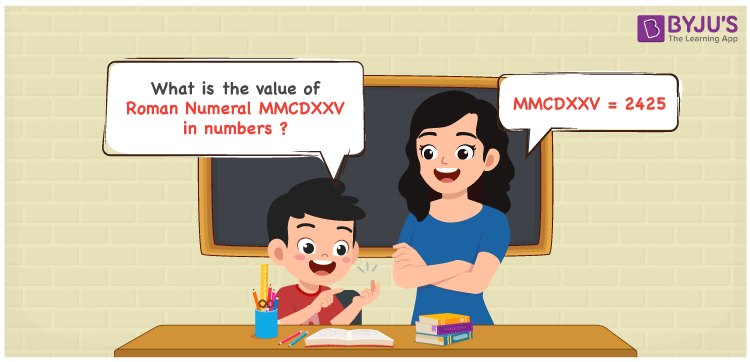MMCDXXV Roman Numerals is 2425. In this article, MMCDXXV is split using the addition operation and transformed to numbers in an interactive manner. For a stronghold on these Maths concepts, the materials at BYJU’S are very useful for the students. Roman numerals articles provide a brief knowledge of the topics so that students get all the details required at one place. Therefore, MMCDXXV can be indicated as 2425 in numbers.

 Number Roman Numeral 2425 MMCDXXV## How to Write MMCDXXV Roman Numerals in Numbers?

Learn the transformation of MMCDXXV to numbers by reading the expansion provided below.

MMCDXXV = M + M + (D – C) + X + X + V

MMCDXXV = 1000 + 1000 + (500 – 100) + 10 + 10 + 5

MMCDXXV = 25425

## Video Lesson on Roman Numerals## Frequently Asked Questions on MMCDXXV Roman Numerals

### Why is the roman numeral of 2425 written as MMCDXXV?

We know that

MMCDXXV = M + M + (D – C) + X + X + V

MMCDXXV = 1000 + 1000 + (500 – 100) + 10 + 10 + 5

MMCDXXV = 2425

### Determine the value of 2500 – 75.

We know that

2500 – 75 = 2425

Hence, the value of 2500 – 75 is 2425 which is written as MMCDXXV.

### What is the remainder we obtain when MMCDXXV is divided by XIX?

We know that

MMCDXXV = 2425

XIX = 19

So the remainder we obtain when MMCDXXV is divided by XIX is XII.